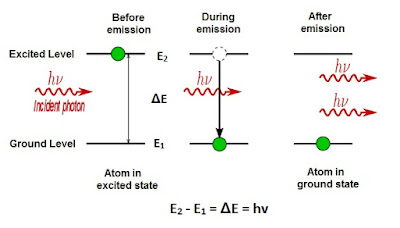## Search This Blog

### Stimulated emission

The concept of stimulated emission was first put forward by Albert Einstein in 1917.

Let us consider two energy levels E1 and E2 in a material. Let us assume that the atom is initially at energy level E2. Also, consider that an electromagnetic wave of frequency v is incident on a given material. This wave has the same frequency as that of the specific material. Therefore, there is a high degree of probability that this wave will force the atom to undergo a change from energy level E2 to energy level E1. In this case, the energy difference E2 - E1 is received in the form of an electromagnetic wave which adds to the incident wave. Here, it is to be noted that the atom is already in the excited state E2. Before it could come to the ground state, due to the spontaneous emission process, if it is irradiated with a photon, whose energy is exactly equal to E2 - E1. The incident photon will stimulate the excited atom to emit one photon of exactly the same energy, as that of the incident photon. Thus, two photons will be emitted in this process. This phenomenon is known as Stimulated Emission.Note: The most remarkable feature of the stimulated emission is both the emitted photons will have the same frequency, phase direction and polarization, as that of the incident photon. So, in this process, we give the input of one incident photon and obtain the two photons identical in all respects as an output. Thus, amplification of radiation takes place by the stimulated process.